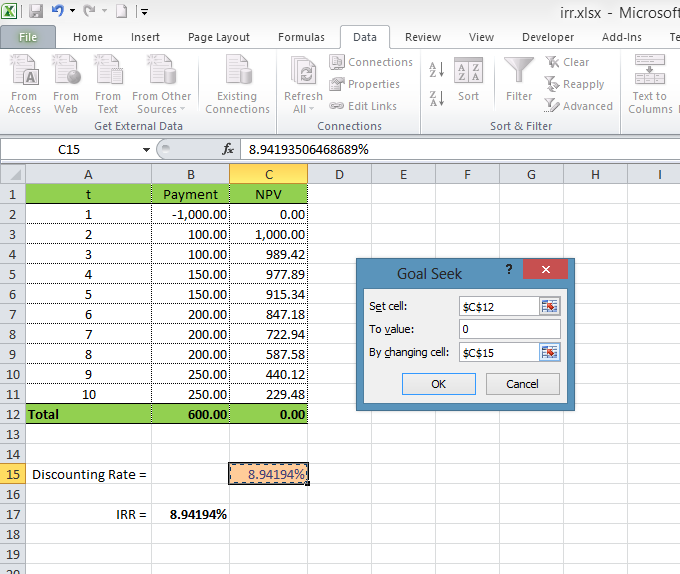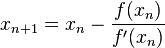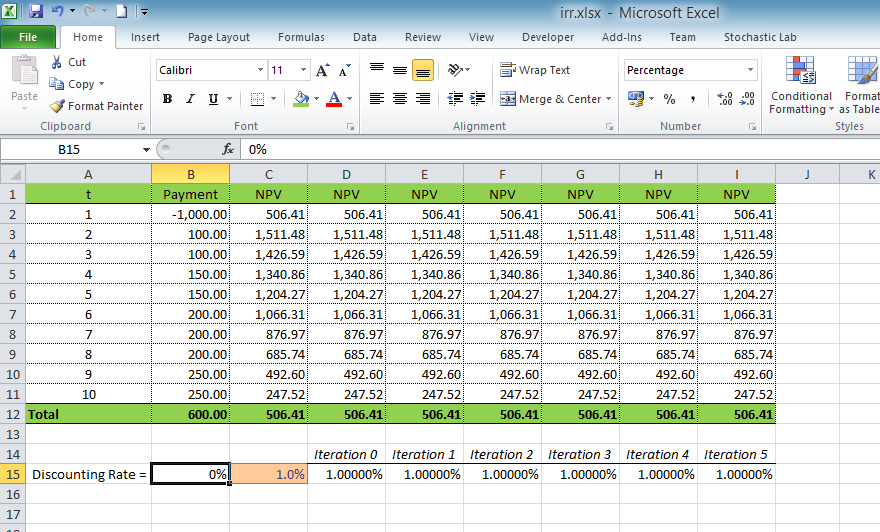# Calculating Internal Rate of Return (IRR) using Excel

Internal Rate of Return is another method used for cash flow valuation. IRR is a discount rate, whereby NPV equals to zero. You might use the following Excel function:

`=IRR(payments_range)`

There is no exact and universal formula for the IRR valuation; therefore, only approximate methods are used in practice. For example, you might use the GoalSeek function in Excel. Let's take an example of NPV calculation and try to find the value of discount rate (cell C15), so that NPV (cell C12) would equal to zero:

•Moreover, you might calculate IRR by using the Newton’s method, where the root of an equation is calculated recursively based on the previous value and the angle tangent of that point:In our case, Х – is a discount rate, f(x) – NPV, and a derivative can be calculated by knowing the value of function for two points.

For more precise results, this method has to be applied several times. Let’s add more columns for calculating several iterations:

•Above picture shows the several iterations for calculating NPC at discount rate of 1%.

Let's specify the formula for calculating the discount rate. First of all, we need to calculate IRR for iteration №0. For doing this, we need to know the start value (for example, 1% - cell C15) and NPV (cell C12). Moreover, for calculating the derivative we need to know more values: let's assume that NPV is the total sum of payments (cell B12) if the discount rate is 0% (cell B15). Then the formula for IRR calculation will be equalled to the cell D15:

`=C15-C12/((C12-B12)/(C15-B15))`

Copy this formula in order to apply it for cells E15:I15, and then you will see that the approximate value of IRR applied to iteration #5 will not differ from the value calculated by means of Excel functions:

•Obviously, it is much easier to use the IRR formula in Excel, however in this article this calculation method is shown as an example. The principle of this method can be used in practice, especially in such situations where the use of macro commands or GoalSeek functions is not possible.